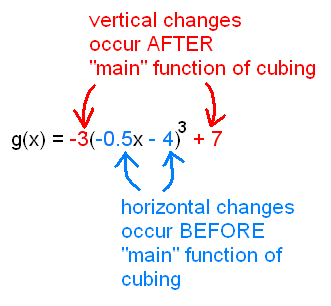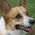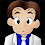## Sunday, February 6, 2011

### Function Transformations Nitty Gritties

I've been racking my brain about how I am going to break down to my Precalc kids the procedures for how to analyze the various transformations involved in a function that looks complicated like g(x) = -3(-0.5x - 4)^3 + 7... Transformations as in, when you compare g(x) against its parent function, f(x) = x^3, what types of shifts and scaling and reflections had resulted in this new function g. This topic is always really difficult for kids, because I am sure that when a regular kid looks at the many numbers inside a complicated transformed equation, all of the number just melt together and seem to be indistinguishable.

Well, I think I've got the big-picture concept finally nailed down to one picture. I hope this is children-safe. It's sort of like an input/output diagram of a function box, but annotated.So, basically, the way I see it, some transformations happen outside of the parentheses (such as multiplying by -3 and adding 7, in my example function g...see below for color-coding) because -- if you consider PEMDAS -- they occur AFTER the "main" function of cubing has already occurred. At that point, it's too late to be affecting x-values, so you're naturally affecting only y-values and causing vertical changes. And since kids already know that higher coefficient = steeper graph, it shouldn't be difficult for them to figure out that this means -3 does the vertical flip / vertical stretch by scale of 3, and 7 does the vertical shift.On the contrary, (if you again consider PEMDAS,) transformations that occur inside the parentheses had occurred BEFORE the "main" function of cubing occurred. So that means they were operating only on the x-values and therefore only resulted in horizontal changes. Again, most kids can figure out that a fractional coefficient of 0.5 makes the graph flatter, so it shouldn't be hard to make the leap that 0.5 = 1/2 stretches it horizontally by a factor of 2. (Since horizontal stretching makes a graph flatter, whereas a horizontal compression would have made the graph look skinnier/steeper, and therefore would have had a coefficient greater than 1 on the inside.) And -- keeping with the theme of "inside" operations affecting only the x-values -- the negative sign on the -0.5 necessarily makes the graph flip horizontally across the y-axis.

The only complication that remains to be explained is why the horizontal shift is 8 to the left, rather than something immediately visible inside the equation, such as 4 units. The way I've always thought about it, special things happen at f(0) for most parent functions. In order for you to capture that same special point on the transformed graph, you have to find the special value x that would result in you still evaluating zero in the "main" function (in this case, by the time you reach the actual cubing operation, the stuff inside the cube would need to be zero in order for you to observe the same special behavior). So, by setting -0.5x - 4 = 0 we get x = -8, or our special point (and every other point from the old graph) has apparently shifted 8 units to the left!

...Obviously, this reliance on order-of-operations can be translated (harhar) to other parent functions as well. Here are some examples, with color coding to show which operations come before and after the "main" function operation.(Of course, my juniors will probably have trouble with simply articulating PEMDAS in abstract algebraic form. Whenever that happens, I make them actually plug a value into x, in order for them to write down all the steps of what operation happened, in which order, before they arrived at the result.)

What do you think? Is this explanation child-proof, or still way too complicated? I don't want to be giving the kids a bunch of blind rules to memorize that they don't understand, especially because there are already so many parent functions that they are keeping track of. I also made a long GeoGebra exploration activity that will cover most of this material without any lecturing on my part, but I'll blog about it only after I figure out how well my kids can absorb the stuff through the scaffolded (but long!) activity. Wish me luck!!

1.In Alg II, I teach almost all graphing as graping by translating, and they learn "what is inside changes signs, what is outside stays the same." So if you have y = (x - 2)^2 + 3, you know your new origin will be at (2, 3). Want to throw in y = 3(x - 2)^2 + 3? Well, that makes the parent graph y = 3x^2. So take your original t-chart {(-2, 4)(-1, 1)(0,0)(1, 1)(2,4)} and multiply all the y's by 3 and graph these points from your new "origin." Want to get really crazy? (and I mostly leave this for trig) y = 3(2x-2)^2 + 3. Make it look pretty: y = 3(2(x-1))^2 + 3. Find the vertex: (1, 2). Find your parent: y = 3(2x)^2. Multiply the y's of the original by 3. Since 2 is inside the parenthesis, do the opposite--divide your x values by 2. So now you have (-1, 12) (-.5, 3)(0,0) (.5, 3), (1, 12) that you will translate to (1, 2).

Maybe it seems like "just a bunch of blind rules" but we discuss the "why" when we first introduce it (with absolute value), then after that it's a piece o' cake.

2.This looks really good and the combination of explanatory notes and an exploratory activity should help to lock in the learning.

One minor hiccup came to mind: some students could get momentarily lost with h(x) and may need to see it re-written as

h(x) =

4*( 1 ) - 2
(------)
(-5x+1 )

http://www.wolframalpha.com/input/?i=h%28x%29%3D4*%281%2F%28-5x%2B1%29%29-2

to be able to successfully apply the PEMDAS model you’ve built for them. But I think that that is why h(x) is a great example to include in the lesson.

3.Sam has nice sequence for this topic with very detailed worksheets/notes. It might give you some ideas.

http://samjshah.com/2009/04/23/function-transformations-2/

4.@Mr. H: thanks! I've definitely spent a good while carefully poring over those handouts yesterday before writing my lesson. I liked his approach of making kids plot each sub-transformation one at a time. It's a good in-class practice sort of thing once kids have a basic grasp of the patterns, but I wanted the exploration part to be computer-based, so I ended up whipping up my own version instead of just using his.

My kids are also not starting completely from scratch with this material. They already can efficiently graph things like
f(x) = -3(x - 2)^2 - 6 using transformational ideas a la what Meg described above, so making the exploratory part on the computer has the added benefit of speeding along the concept-building.

@Meg Sam Shah's worksheets (see Mr. H's link above) use the same method of factoring to "make it pretty" as you suggest. But, how do you explain to your kids why we "do the opposite" on the inside, and why it's necessary to "make it pretty"? That's part of what I've always struggled with.

@Paul Got it. Thanks for looking out. Rational functions are tricky because it's the only one where kids can't think in terms of what's "inside" vs. "outside"... Think that's the one where the understanding of order of ops will really come into play.

5.Whipping up a quick GeoGebra applet with adjustable transformation parameters really brings it alive for some students.

6.One of the ways that I attack the problem of moving in the opposite direction is to describe the transformation as happening not to the graph, but rather to the axes. So, for example, y = (x-2)^2 will mean graph x^2 but shift the axes to the left by 2. Of course, one might counter that now the vertical shifts are moving in the wrong direction, but this is only because we choose to put the shift on the wrong side. For instance, y = x^2 + 2 could be written as y - 2 = x^2 and then it all makes sense: graph x^2 and move the axes down 2.

1.This is a fantastic explanation! I really like it!

7.Notice that @glsr's system works for stretches and squishes as well.

y = sin( x/3 ) is a STRETCH in x

y = 3 sin(x) ---> (y/3) = sin(x)

this is a STRETCH in y.

8.I like the idea of the axes being stretched/squished/translated. I'm going to use it. Thanks!

9.Hi there,
I am teaching transformations in algebra 2 and I came across your blog in my research. It's almost a year later, did you find this technique worked well? What are you doing differently this year? My kids are really struggling with these (and so have past students) so it's my goal to find some good techniques on this!
Jessie

10.Hi Jessie,

It worked ok for me but not great. This year the curriculum is different so I cannot really say if there is one thing I am changing, but my colleague says that she finds it much easier to first teach quadratic transformation, then sinusoidal transformation, then general transformation. Move from concrete to abstract helps the kids understand.

Mimi